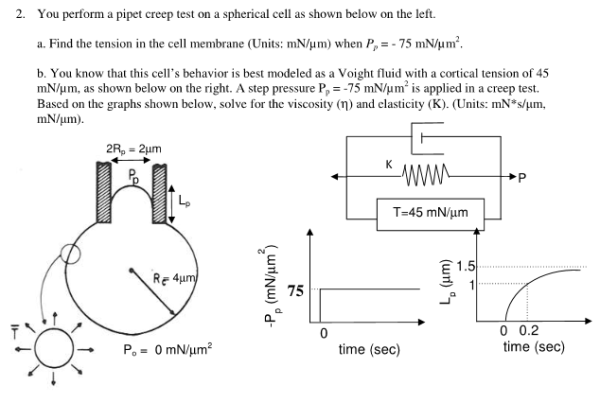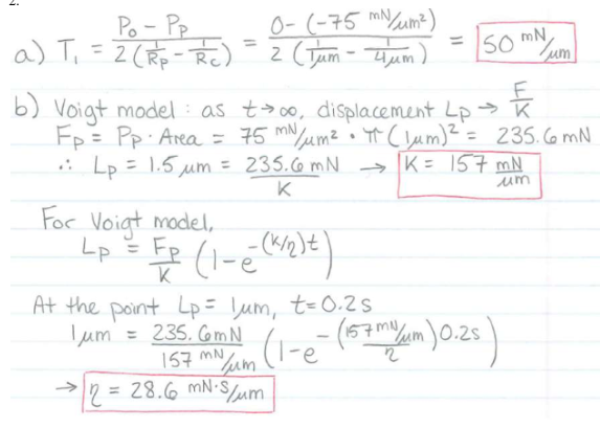You perform a pipet creep test on a spherical cell as shown below on the left. a) Find the tension in the cell membrane when Pp=-75 mN/um^2. b) You know that this cell's behavior is best modled as a Voight fluid with a cortical tension of 45 mN/um, as shown below on the right. A step pressure Pp=-75 mN/um^2 is applied in a creep test. Based on the graphs shown below, solve for the viscosity and elasticity.You perform a pipet creep test on a spherical cell as shown below on the left. a) Find the tension in the cell membrane when Pp=-75 mN/um^2. b) You know that this cell's behavior is best modled as a Voight fluid with a cortical tension of 45 mN/um, as shown below on the right. A step pressure Pp=-75 mN/um^2 is applied in a creep test. Based on the graphs shown below, solve for the viscosity and elasticity.

biomechanics page 1 biomechanics biomechanics biomechanics biomechanics biomechanics biomechanics biomechanics biomechanics page 2